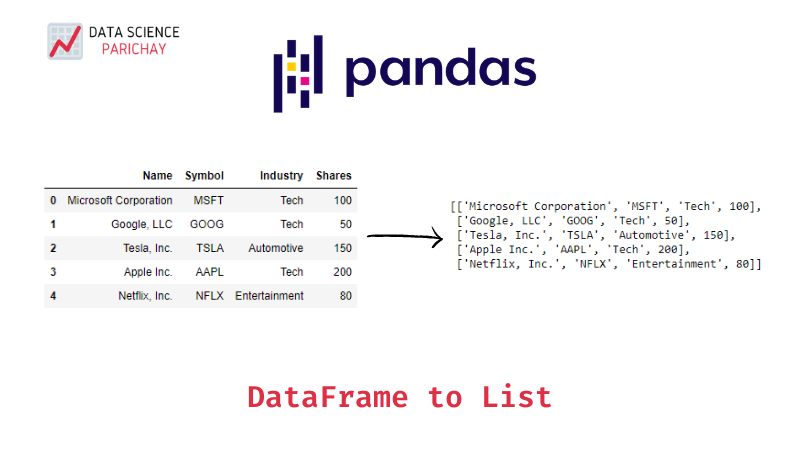# Pandas DataFrame to a List in Python

Pandas dataframes are great for manipulating data. But, at times it might happen that you’d rather have the data as a list (or more precisely, a list of lists). In this tutorial, we’ll look at how to convert a pandas dataframe to a python list.

There are multiple ways to get a python list from a pandas dataframe depending upon what sort of list you want to create. To quickly get a list from a dataframe with each item representing a row in the dataframe, you can use the `tolist()` function like `df.values.tolist()`

However, there are other ways as well. You can create a list with each item representing a dataframe column. Or, you can create something very specific based on your requirements. Let’s look at some of the different use cases with examples.

First, let’s create a dataframe of a sample stock portfolio that we’ll be using throughout this tutorial.

``````import pandas as pd

data = {
'Name': ['Microsoft Corporation', 'Google, LLC', 'Tesla, Inc.',\
'Apple Inc.', 'Netflix, Inc.'],
'Symbol': ['MSFT', 'GOOG', 'TSLA', 'AAPL', 'NFLX'],
'Industry': ['Tech', 'Tech', 'Automotive', 'Tech', 'Entertainment'],
'Shares': [100, 50, 150, 200, 80]
}

df = pd.DataFrame(data)
print(df)``````

Output:

``````                    Name Symbol       Industry  Shares
0  Microsoft Corporation   MSFT           Tech     100
1            Google, LLC   GOOG           Tech      50
2            Tesla, Inc.   TSLA     Automotive     150
3             Apple Inc.   AAPL           Tech     200
4          Netflix, Inc.   NFLX  Entertainment      80``````

The following are some of the ways to get a list from a pandas dataframe explained with examples.

As mentioned above, you can quickly get a list from a dataframe using the `tolist()` function.

``````ls = df.values.tolist()
print(ls)``````

Output

📚 Data Science Programs By Skill Level

Introductory

Intermediate ⭐⭐⭐

🔎 Find Data Science Programs 👨‍💻 111,889 already enrolled

Disclaimer: Data Science Parichay is reader supported. When you purchase a course through a link on this site, we may earn a small commission at no additional cost to you. Earned commissions help support this website and its team of writers.

``[['Microsoft Corporation', 'MSFT', 'Tech', 100], ['Google, LLC', 'GOOG', 'Tech', 50], ['Tesla, Inc.', 'TSLA', 'Automotive', 150], ['Apple Inc.', 'AAPL', 'Tech', 200], ['Netflix, Inc.', 'NFLX', 'Entertainment', 80]]``

In the above example, `df.values` returns the numpy representation of the dataframe `df` which is then converted to a list using the `tolist()` function. You can see that we get a list of lists with each item in the list representing a row in the dataframe.

You can also use `tolist()` function on individual columns of a dataframe to get a list with column values.

``````# list with each item representing a column
ls = []
for col in df.columns:
# convert pandas series to list
col_ls = df[col].tolist()
# append column list to ls
ls.append(col_ls)
# print the created list
print(ls)``````

Output

``````[['Microsoft Corporation', 'Google, LLC', 'Tesla, Inc.', 'Apple Inc.', 'Netflix, Inc.'], ['MSFT', 'GOOG', 'TSLA', 'AAPL', 'NFLX'], ['Tech', 'Tech', 'Automotive', 'Tech', 'Entertainment'], [100, 50, 150, 200, 80]]
``````

In the above example, we iterate through each column of the dataframe which is converted to a list and then appended to `ls`. You can see that here we get a list of lists with each item in the list representing a column in the dataframe.

You can also create a list by iterating through the rows of the dataframe.

``````ls = []
# iterate over the rows
for i, row in df.iterrows():
# create a list representing the dataframe row
row_ls = [row['Name'], row['Symbol'], row['Industry'], row['Shares']]
# append row list to ls
ls.append(row_ls)

print(ls)``````

Output

``````[['Microsoft Corporation', 'MSFT', 'Tech', 100], ['Google, LLC', 'GOOG', 'Tech', 50], ['Tesla, Inc.', 'TSLA', 'Automotive', 150], ['Apple Inc.', 'AAPL', 'Tech', 200], ['Netflix, Inc.', 'NFLX', 'Entertainment', 80]]
``````

In the above example, we use the pandas dataframe `iterrows()` function to iterate over the rows of `df` and create a list with row values which gets appended to `ls`. You can see that we get a list of lists with each item in the list representing a row in the dataframe like we saw in the example with the `tolist()` function.

This method also allows you the flexibility to create specific lists based on your requirements. For instance, from the above dataframe if you want to create a list of lists with only the stock symbol and its respective share count you can easily do it by keeping only those fields.

``````ls = []
# iterate over the rows
for i, row in df.iterrows():
# create a list representing the dataframe row
row_ls = [row['Symbol'], row['Shares']]
# append row list to ls
ls.append(row_ls)

print(ls)``````

Output

``````[['MSFT', 100], ['GOOG', 50], ['TSLA', 150], ['AAPL', 200], ['NFLX', 80]]
``````

Here, we get a list of lists with each item having the stock symbol and the respective shares count in the portfolio.

With this, we come to the end of this tutorial. The code examples and results presented in this tutorial have been implemented in a Jupyter Notebook with a python (version 3.8.3) kernel having pandas version 1.0.5

•